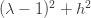# Symplectic Integrators

Posted on the 12 December 2013 by Ccc1685 @ccc1685

Dynamical systems can be divided into two basic types: conservative and dissipative.  In biology, we almost always model dissipative systems and thus if we want to computationally simulate the system almost any numerical solver will do the job (unless the problem is stiff, which I’ll leave to another post). However, when simulating a conservative system, we must take care to conserve the conserved quantities. Here, I will give a very elementary review of symplectic integrators for numerically solving conservative systems.

The prime example is a Hamiltonian system, where energy is conserved. More precisely, the symplectic form, which includes orientation as well as magnitude, is preserved. Consider the simple harmonic oscillator with the Hamiltonian:Hamilton’s equations areIf we were to blindly use Euler’s method to numerically integrate these equations we would getwhere h is the step size. We can rewrite this asWe assess stability of this numerical scheme by setting, in other words, compute the eigenvalues of the matrix system. The characteristic equation,, has solutions. The magnitude of the largest eigenvalue is, which is greater than one so the system is unstable. Hence, the Euler scheme will never work for a Hamiltonian system no matter how small you make h.  Eventually, it will blow up.

This can be fixed with an extremely simple tweak to preserve the symplectic form and naturally enough this is called a symplectic integrator:The only difference is that you update the p equation using the updated value of q.  In fact, if you were to absentmindedly code this system you could easily accidentally discover the symplectic integrator. However,  you wouldn’t know why it worked or even that it may not always work and that is a very dangerous thing.

If we substitute, we now get the systemwhich has solutions, which are always magnitude one.  Hence, the symplectic integrator is stable and the energy will remain bounded.  The symplectic scheme generalizes to nonlinear Hamiltonian equations as well. The trick is just that you update each equation sequentially using all the information up to that point in time.

This idea can generalize to PDEs as well.  Consider the advection equationThis equations conserves. A simple finite difference scheme on a spatial lattice of N points isWe can asses stability by substituting in, which gives, whose magnitude is larger than one.  However, if we use the symplectic schemeWe arrive atwhich has magnitude of one and the scheme is thus stable.

See more